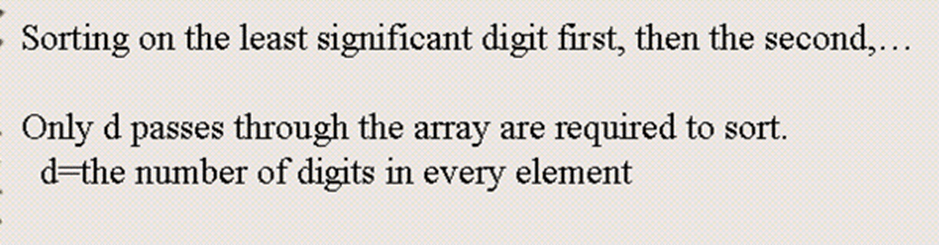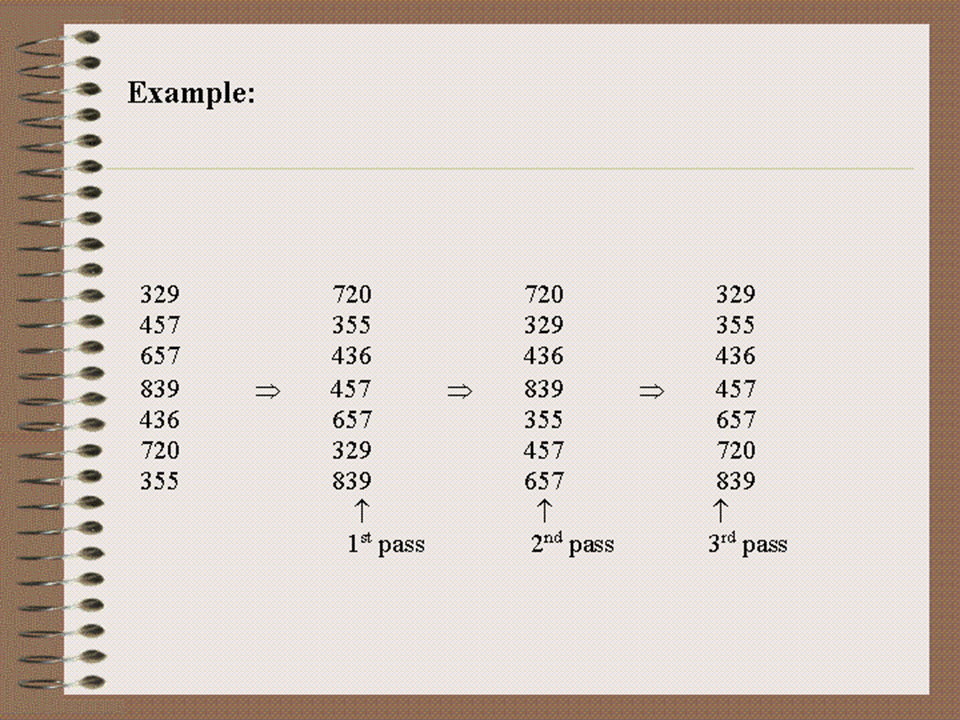• Assumes that all elements in an array have same number of digits## Running Time

• d is constant
• Running time of radix-sort = Running time of stable sort * d
• Use count sort as sorting technique = O(n)
• d* O(n) = O(n)

Radix sort is also linear time sorting technique